# Systems Of Linear Equations With One Solution (3 Ways To Tell)

When working with systems of linear equations, we often see infinitely many solutions or one at all.  However, it is also possible that a linear system will have exactly one solution.

So, when does a system of linear equations have one solution?  A system of two linear equations in two variables has one solution when the two lines have different slopes. From an algebra standpoint, this means that we get a single value when solving the system. Visually, the lines intersect exactly once on a graph, since they have different slopes.

Of course, a system of three equations in three variables has one solution if there is exactly one point where all three planes intersect.

In this article, we’ll talk about how you can tell that a system of linear equations has one solution.  We’ll also look at some examples of linear systems with one solution in 2 variables and in 3 variables.

Let’s begin.

## Systems Of Linear Equations With One Solution

A system of linear equations can have one solution if there is a single point that makes every equation in the system true.  This means that there is one point that can satisfy all of the equations at the same time.

The image below summarizes the 3 possible cases for the solutions for a system of 2 linear equations in 2 variables.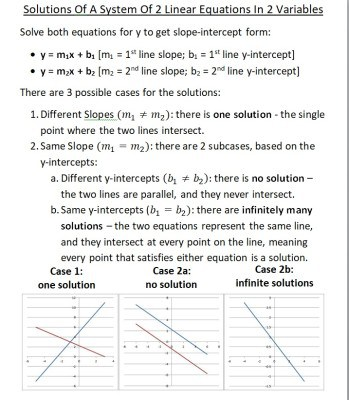A system of 2 linear equations in 2 variables has one solution when the two lines have different slopes.

A system of equations in 2, 3, or more variables can have one solution.  We’ll start with linear equations in 2 variables with one solution.

## When Does A Linear System Have One Solution? (System Of Linear Equations In 2 Variables)

There are a few ways to tell when a linear system in two variables has one solution:

• Solve the system – if you solve the system and get a single equation (such as x = 2 and y = 5), then there is one solution.
• Look at the graph – if the two lines have different slopes (they intersect exactly once), then there is one solution to the system.
• Look at the slope and y-intercept – solve both equations for y to get slope-intercept form, y = mx + b.  If the two equations have different slopes, then the two lines are different and are not parallel, so there is only one solution (you can get a refresher on how to tell when two lines are parallel in my article here).

We’ll look at some examples of each case, starting with solving the system.

### Solving A Linear System With One Solution

When we solve a linear system with one solution, we will get a result that gives us a single value for x and a single value for y.  For example, after we solve, we will get something like x = 2 and y = 5.

Let’s take a look at some examples to see how this can happen.

#### Example 1: Using Elimination To Show A Linear System Has One Solution

Let’s say we want to solve the following system of linear equations:

• 2x + 4y = 3
• -6x – 8y = 11

We will use elimination to solve.  Let’s try to eliminate the “x” variable.

We begin by multiplying the first equation by 3 to get:

• 3(2x + 4y) = 3(3)  [multiply the first equation by 3 on both sides]
• 6x + 12y = 9  [distribute the 3 through parentheses]

Now we add this modified equation to the second one:

6x + 12y = 9

+

-6x – 8y = 11

___________

0x + 4y = 20

This implies y = 5.  Substituting y = 5 into the first equation gives us:

• 2x + 4y = 3
• 2x + 4(5) = 3
• 2x + 20 = 3
• 2x = -17
• x = -8.5

So the solution is (-8.5, 5).  We can test this by substituting x = -8.5, y = 5 into both of the original equations to make sure we get the right values.

Since there is only one ordered pair that makes both equations true, there is only one solution to this system of linear equations. The graph below confirms that the lines have different slopes and intersect exactly once.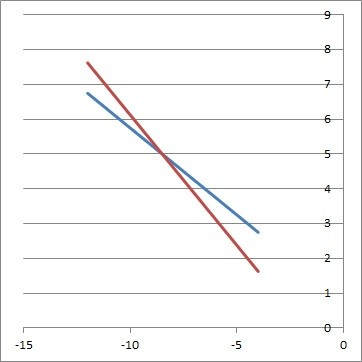The two lines 2x + 4y = 3 (blue) and -6x – 8y = 11 (red) have different slopes, so they intersect at one point, and there is one solution to the linear system.

#### Example 2:  Using Substitution To Show A Linear System Has One Solution

Let’s say we want to solve the following system of linear equations:

• y = 2x + 5
• 3x + 4y = 9

We will use substitution to solve.  We’ll substitute the y from the first equation into the y in the second equation:

• 3x + 4(2x + 5) = 9  [substitute y = 2x + 5 from the first equation]
• 3x + 8x + 20 = 9  [distribute 4 through parentheses]
• 11x = -11  [combine like terms on opposite sides]
• x = -1  [divide by 11 on both sides]

Now we can substitute x = -1 into the first equation to get:

• y = 2(-1) + 5
• y = -2 + 5
• y = 3

So the solution is (-1, 3).

Since there is only one ordered pair that makes both equations true, there is only one solution to this system of linear equations. The graph below confirms that the lines have different slopes and intersect exactly once.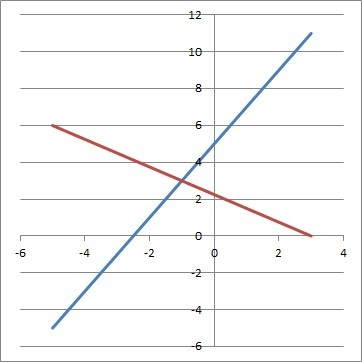The two lines y = 2x + 5 (blue) and 3x + 4y = 9 (red) have different slopes, so they intersect at one point, and there is one solution to the linear system.

### Looking At The Graph Of A Linear System With One Solution

When we graph a linear system with one solution, we will get two different lines that are not parallel (that is, they will have different slopes).

Let’s take a look at some examples to see how this can happen.

#### Example 1: Graph Of Two Intersecting Lines From A Linear System With One Solution

Let’s graph the following system of linear equations:

• y = 2x + 4
• y = -4x – 2

The lines have different slopes (2 and -4), as you can see in the graph below: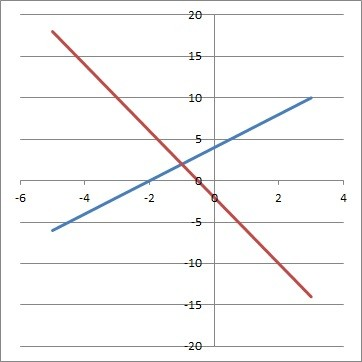The two lines y = 2x + 4 (blue) and y = -4x – 2 (red) have different slopes, so they intersect at one point, and there is one solution to the linear system.

Since the slopes are different, the lines will intersect at exactly one point.  This means that there a single ordered pair (x, y) = (-1, 2) that is a solution to the linear system we started with (this is the only point that lies on both lines).

#### Example 2:  Graph Of Two Intersecting Lines From A Linear System With One Solution

Let’s graph the following system of linear equations:

• x = 2
• y = -3

The first line has an undefined slope (it is a vertical line), and the second line has a slope of zero.  This tells us that the slopes are different, as you can see in the graph below: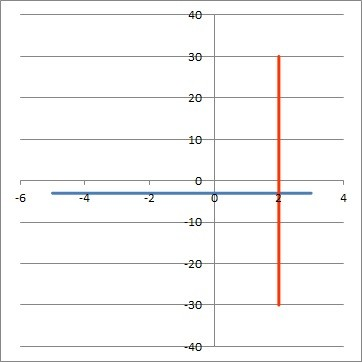The two lines y = -3 (blue) and x = 2 (red) have different slopes, so they intersect at one point, and there is one solution to the linear system.

Since the slopes are different, the lines will intersect at exactly one point.  This means that there a single ordered pair (x, y) = (2, -3) that is a solution to the linear system we started with (this is the only point that lies on both lines).

### Looking At The Slope & Y-Intercept To Show There Is One Solution To A System Of Two Linear Equations

When we solve a linear equation for y, we get slope-intercept form.  If we do this for both equations in a linear system, we can compare the slope and y-intercept.

If the two slopes are different, then we have two different lines that are not parallel, meaning they intersect at one point and there is exactly one solution to the linear system.

Let’s take a look at some examples to see how this can happen.

#### Example 1: Comparing Slope & Y-Intercept To Show There Is One Solution To A System Of Two Linear Equations

Let’s say we have the following system of linear equations:

• 4x = – 2y + 8
• 3y = -12x + 6

We will solve for y in both equations to get slope-intercept form, y = mx + b.

Solving the first equation for y, we get:

• 4x = – 2y + 8
• 4x + 2y = 8  [add 2y to both sides]
• 2y = -4x + 8  [subtract 4x from both sides]
• y = -2x + 4  [divide by 2 on both sides]

Solving the second equation for y, we get:

• 3y = -12x + 6
• y = -4x + 2  [divide by 3 on both sides]

So, the two equations in slope-intercept form are:

• y = -2x + 4
• y = -4x + 2

Since these two equations have different slopes (-2 and -4), we know that they are two different lines that are not parallel.  Since the lines intersect exactly once, there is one solution to the system: the point (-1, 6) which lies on both lines.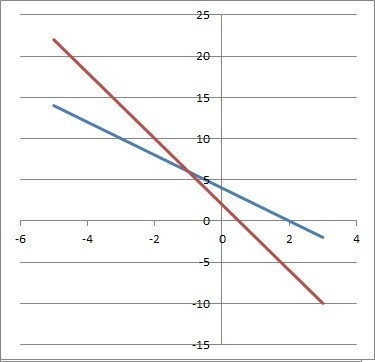The two lines y = -2x + 4 (blue) and y = -4x + 2 (red) have different slopes, so they intersect at one point, and there is one solution to the linear system.

#### Example 2: Comparing Slope & Y-Intercept To Show There Is One Solution To A System Of Two Linear Equations

Let’s say we have the following system of linear equations:

• 30x = 6y – 18
• 4y – 32x + 5 = 13

We will solve for y in both equations to get slope-intercept form, y = mx + b.

Solving the first equation for y, we get:

• 30x = 6y – 18
• 30x + 18 = 6y  [add 18 to both sides]
• 5x + 3 = y  [divide by 6 on both sides]

Solving the second equation for y, we get:

• 4y – 32x + 5 = 13
• 4y – 32x = 13 – 5  [subtract 5 from both sides]
• 4y – 32x = 8  [combine like terms: constants]
• 4y = 32x + 8  [add 32x to both sides]
• y = 8x + 2  [divide by 4 on both sides]

So, the two equations in slope-intercept form are:

• y = 5x + 3
• y = 8x + 2

Since these two equations have different slopes (5 and 8), we know that they are two different lines that are not parallel.  Since the lines intersect exactly once, there is one solution to the system: the point (1/3, 14/3) which lies on both lines.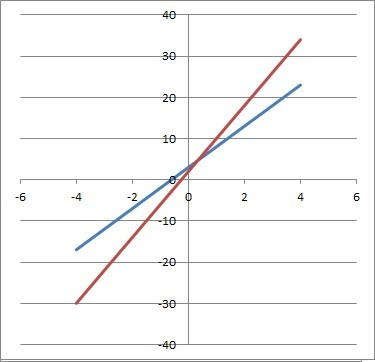The two lines y = 5x + 3 (blue) and y = 8x + 2 (red) have different slopes, so they intersect at one point, and there is one solution to the linear system.

## How To Create A System Of Linear Equations With One Solution

To create a system of linear equations with one solution, we can take a couple of approaches:

• One method is to start by choosing values “a” and “b” for any expression ax + by.  Next, choose values of x and y for a solution (x, y).  Then, calculate the value of ax + by, which is the value of c.  The equation ax + by = c is our first equation.  Repeat the steps for another pair of values “e” and “f”, the same solution (x, y), and find a value of “g” to get the second equation ex + fy = g.
• Another method is to graph the solution (x, y) and then draw two lines with different slopes that go through (x, y).  Find the equation of both lines and you will have a system with a solution of (x, y).

Let’s try each method in turn.

### Example  1: Create A System Of Linear Equations With One Solution

Let’s choose values of a = 2 and b = 3.  Then our equation will look like:

• ax + by = c
• 2x + 3y = c

All we need to do is find c for a given point (x, y).  Let’s choose x = 1, y = 4 to get:

• 2x + 3y = c
• 2(1) + 3(4) = c
• 2 + 12 = c
• 14 = c

So our first equation is

• 2x + 3y = 14 (a = 2, b = 3, c = 14)

Now we repeat the steps for our second equation.

Let’s choose values of e = 5 and f = 4.  Then our equation will look like:

• ex + fy = g
• 5x + 4y = g

All we need to do is find g for the same point x = 1, y = 4:

• 5x + 4y = g
• 5(1) + 4(4) = g
• 5 + 16 = g
• 21 = g

So our second equation is

• 5x + 4y = 21 (e = 5, f = 4, g = 21)

Then the system of linear equations is:

• 2x + 3y = 14
• 5x + 4y = 21

We can solve by substitution or elimination to verify that the solution is the point (1, 4).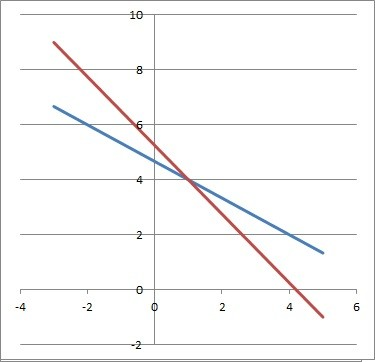The two lines 2x + 3y = 14 (blue) and 5x + 4y = 21 (red) have different slopes, so they intersect at one point, and there is one solution to the linear system.

### Example 2: Create A System Of Linear Equations With One Solution

Let’s say we want a linear system with a solution of (3, 7).  We will draw two lines that go through this point:

[graph of 2 lines below, and point (3, 7)]

The first line has a slope of 4 and a y-intercept of -5.  Its equation is y = 4x – 5.

The second line has a slope of -2 and a y-intercept of 13.  Its equation is y = -2x + 13.

So, our system of linear equation is:

• y = 4x – 5
• y = -2x + 13

We can solve by substitution or elimination to verify that the solution is the point (3, 7).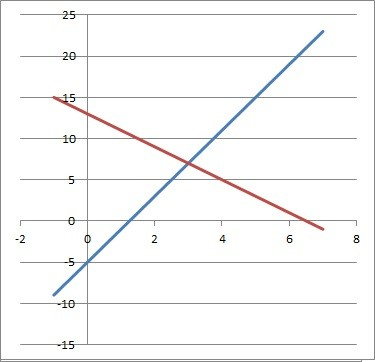The two lines y = 4x – 5 (blue) and y = -2x + 13 (red) have different slopes, so they intersect at one point, and there is one solution to the linear system.

## System Of Linear Equations In Three Variables With One Solution

A system of equations in 3 variables will have one solution if there is a single point where the 3 planes all intersect.  Here is an example:

• x + y + z = 1
• x + y + 2z = 2
• x + 2y + 3z = 3

We can easily eliminate the variable x by subtracting equations:

• y + 2z = 2  [subtract first equation from third]
• z = 1  [subtract first equation from second]

Using z = 1 in the equation y + 2z = 2 gives us y = 0.

Using z = 1 and y = 0 in the first equation gives us x = 0.

So, the solution is the ordered triple (0, 0, 1).

## When Does A System Of Linear Equations Have A Solution?

A system of linear equations in two variables has a solution when the two lines intersect in at least one place.

• If the two lines have the same slope and the same y-intercept, then the two equations are equivalent, and they represent the same line (so there are infinitely many solutions, since every point on the line is a solution).
• If the two lines have different slopes, then they intersect at exactly one point.

## When Does A System Of Linear Equations Have No Solution?

A system of two linear equations in two variables has no solution when the two lines are parallel.

From an algebra standpoint, this means that we get a false equation when solving the system.

Visually, the lines never intersect on a graph, since they have the same slope but different y-intercepts.

## Conclusion

Now you know when a system of linear equations has one solution.  You also know what to look out for in terms of the slope, y-intercept, and graph of lines in these systems.

You might also find it helpful to read my article on systems of linear equations with infinite solutions.